Probability Concepts | IFT World
IFT Notes for Level I CFA® Program
IFT Notes for Level I CFA® Program

# Part 4

## 6. Bayes’ Formula

Bayes’ formula is a rational method for updating or adjusting the probability of an event based on new information. According to Bayes’ formula, the updated probability of an event given new information is: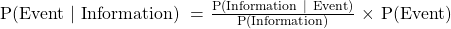Example

Consider a factory that has three assembly lines. The percentage of output produced at each assembly line is as follows: Line A = 45%, Line B = 35%, Line C = 20%. The output defective from each line is estimated to be 3%, 5%, and 4%, respectively. Given that the product is defective, what is the probability that it came from Line C?

Solution:

When dealing with questions related to Bayes’ formula, the first step is to reproduce the information in probability notation:

P(Line A) = 0.45; P(Not Line A) = 0.55

P(Line B) = 0.35; P(Not Line B) = 0.65

P(Line C) = 0.20; P(Not Line C) = 0.80

P(Defective | Line A) = 0.03, P(Defective | Line B) = 0.05, P(Defective | Line C) = 0.04

P(Defective) = 0.45 x 0.03 + 0.35 x 0.05 + 0.20 x 0.04 = 0.039

Next write down the Bayes formula: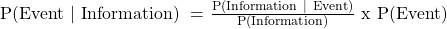We then have to distinguish between the event and the information and plug the relevant values into the formula. In this case, the information is that the product is defective. Hence, the formula can be written as: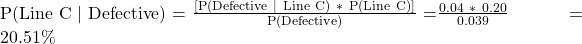## 7. Principles of Counting

In counting, enumeration (counting the outcomes one by one) is the most basic resource. This process is difficult and is prone to error. We will discuss shortcuts and principles of counting, which make the process easier.

Multiplication Rule of Counting

The first of these principles is the multiplication rule. It states that ‘if one task can be done in n1 ways, and a second task, given the first, can be done in n2 ways, and a third task, given the first two tasks, can be done in n3 ways and so on for k tasks, then the number of ways the k tasks can be done is (n1) (n2) (n3)… (nk). So the multiplication rule for counting can be expressed as:

Number of ways of doing k tasks = n1 x n2 x n3…nk

where:

n1= number of ways of doing the first task,

n2= number of ways of doing the second task and so on

Example

Consider a simple example. Suppose we have three steps in an investment decision process. The first step can be done in 2 ways, the second step can be done in 4 ways and the third in 3 ways. In how many ways can the investment decision be made?

Solution:

Following the multiplication rule, there are (2) (4) (3) = 24 ways of making the investment decision.

Notice that there are three groupings in this problem. From each group, only one step can be selected.

Factorial

Another counting principle relates to the assignment of members of a group to an equal number of positions. The number of ways we can assign every member of a group of size n to n slots is n! (read as n factorial) = n (n – 1) (n – 2) (n – 3) … 1. By convention, 0! = 1. The difference between the multiplication rule and factorial is that there is only one group in a factorial. It involves arranging the set of items within the group and the order in which the items are arranged matters. The formula is:

Number of ways of assigning group of size n to n tasks = n!

Example

There are five equity analysts covering five emerging countries. In how many ways can the countries be assigned to the analysts?

Solution:

The total number of ways the assignments can be made = 5! = 120

Labeling

Labeling refers to the number of ways that n items can be labelled with k different labels with n, of the first type, n of the second type, and so on. This can be expressed as:

Number of ways in which n items can be labelled usingExample

A portfolio consists of eight stocks. The goal is to designate four of the stocks as “long-term holds,” three of the stocks as “short-term holds,” and one stock a “sell.” How many ways can these labels be assigned to the eight stocks?

Solution:

Notice that there are eight items (stocks) that are to be labelled in three different ways.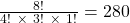Combination

A special case of the labelling is the combination formula. It is the number of ways to choose r objects from a total of n objects, when the order in which the r objects are listed does not matter. This can be expressed as: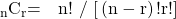where:

n = number of objects

r = number of objects chosen from n objects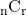= number of ways to choose r objects from n objects where order does not matter

Example

A portfolio manager wants to eliminate four stocks from a portfolio that consists of six stocks. How many ways can the four stocks be sold when the order of the sale is NOT important?

Solution:

Using the formula for combination, we get the number of ways the four stocks can be sold

= 6! / [(6 – 4)! 4!] = 15.

Permutation

Permutation is the number of ways to choose r objects from a total of n objects, when the order in which the r objects are listed does matter. It is expressed as: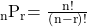where:

n = number of objects

r = number of objects chosen from n objects

Example
Assume that in a portfolio of eight stocks, we decide to sell three stocks. How many ways can we choose three of the eight to sell if the order of sale does matter?
Solution:
Using the formula for permutation, we can find the number of ways to sell three of the eight stocks where order matters: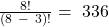Instructor’s Note: given a problem, use the following pointers to identify the correct counting method to apply.

• Factorial: if there is one group of size n and n items/tasks to be assigned, number of ways = n!
• Labeling: used when there are three or more labels. Each item/member of a group must be applied a label.
• Combination: used when there are two groups of a certain size, say n and r. Use combination when the order of choosing r objects from n objects does NOT
• Permutation: used when there are two groups of a certain size, say n and r. Use permutation when the order of choosing r objects from n objects does matter.
• Combination and permutation functions are available on the financial calculator and should be used rather than the formula.